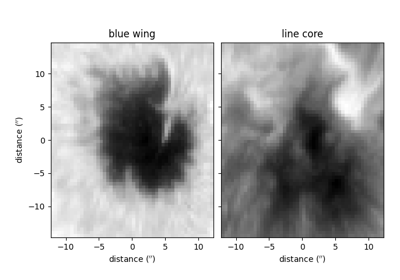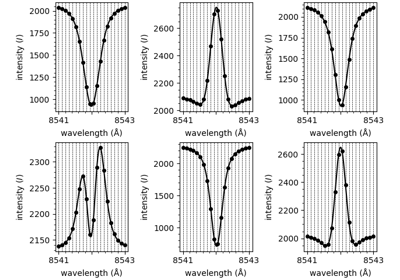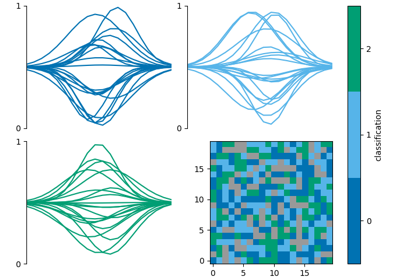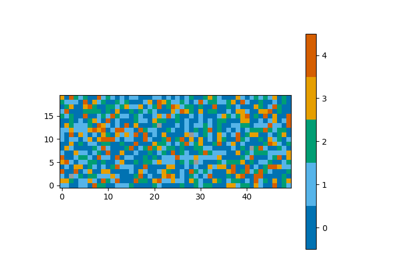# plot_class_map

mcalf.visualisation.plot_class_map(class_map=None, vmin=None, vmax=None, resolution=None, offset=(0, 0), dimension='distance', style='original', cmap=None, show_colorbar=True, colorbar_settings=None, ax=None, data=None)[source]

Plot a map of the classifications.

Parameters:
• class_map (numpy.ndarray[int], ndim=2 or 3) – Array of classifications. If the array is three-dimensional, it is assumed that the first dimension is time, and a time average classification will be plotted. The time average is the most common positive (valid) classification at each pixel.

• vmin (int, optional, default=None) – Minimum classification integer to plot. Must be greater or equal to zero. Defaults to min positive integer in class_map.

• vmax (int, optional, default=None) – Maximum classification integer to plot. Must be greater than zero. Defaults to max positive integer in class_map.

• resolution (tuple[float] or astropy.units.quantity.Quantity, optional, default=None) – A 2-tuple (x, y) containing the length of each pixel in the x and y direction respectively. If a value has type `astropy.units.quantity.Quantity`, its axis label will include its attached unit, otherwise the unit will default to Mm. If resolution is None, both axes will be ticked with the default pixel value with no axis labels.

• offset (tuple[float] or int, length=2, optional, default=(0, 0)) – Two offset values (x, y) for the x and y axis respectively. Number of pixels from the 0 pixel to the first pixel. Defaults to the first pixel being at 0 length units. For example, in a 1000 pixel wide dataset, setting offset to -500 would place the 0 Mm location at the centre.

• dimension (str or tuple[str] or list[str], length=2, optional, default='distance') – If an ax (and resolution) is provided, use this string as the dimension name that appears before the `(unit)` in the axis label. A 2-tuple (x, y) or list [x, y] can instead be given to provide a different name for the x-axis and y-axis respectively.

• style (str, optional, default='original') – The named matplotlib colormap to extract a `ListedColormap` from. Colours are selected from vmin to vmax at equidistant values in the range [0, 1]. The `ListedColormap` produced will also show bad classifications and classifications out of range in grey. The default ‘original’ is a special case used since early versions of this code. It is a hardcoded list of 5 colours. When the number of classifications exceeds 5, `style='viridis'` will be used.

• cmap (str or matplotlib.colors.Colormap, optional, default=None) – Parameter to pass to matplotlib.axes.Axes.imshow. This parameter overrides any cmap requested via the style parameter.

• show_colorbar (bool, optional, default=True) – Whether to draw a colorbar.

• colorbar_settings (dict, optional, default=None) – Dictionary of keyword arguments to pass to `matplotlib.figure.Figure.colorbar()`. Ignored if show_colorbar is False.

• ax (matplotlib.axes.Axes, optional, default=None) – Axes into which the velocity map will be plotted. Defaults to the current axis of the current figure.

• data (dict, optional, default=None) – Dictionary of common classification plotting settings generated by `init_class_data()`. If present, all other parameters are ignored except show_colorbar and ax.

Returns:

im – The object returned by `matplotlib.axes.Axes.imshow()` after plotting class_map.

Return type:

matplotlib.image.AxesImage

`mcalf.models.ModelBase.classify_spectra`

Classify spectra.

`mcalf.utils.smooth.average_classification`

Average a 3D array of classifications.

Notes

Visualisation assumes that all integers between vmin and vmax are valid classifications, even if they do not appear in class_map.

ExamplesWorking with IBIS data

Working with IBIS dataUsing IBIS8542Model

Using IBIS8542ModelCombine multiple classification plots

Combine multiple classification plotsPlot a map of classifications

Plot a map of classifications